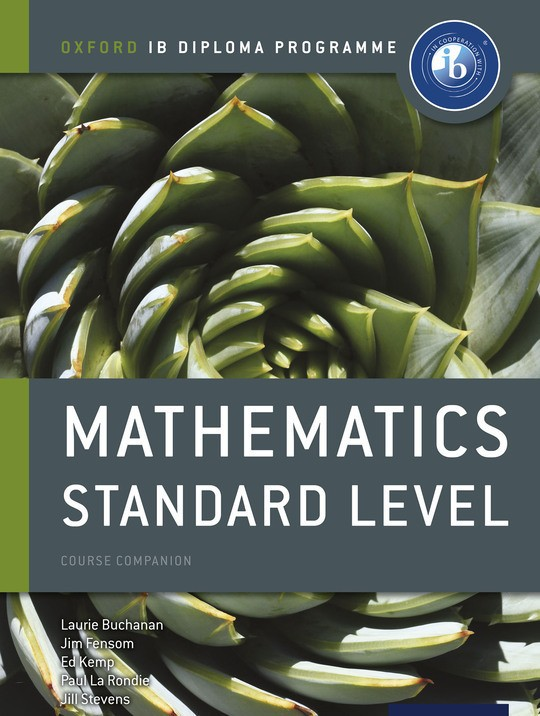\$63.74

# Oxford IB Diploma Programme: Mathematics Standard Level Course Companion

By Paul La Rondie, Ed Kemp, Laurie Buchanan, Jim Fensom, Jill Stevens
US\$ 63.74
The publisher has enabled DRM protection, which means that you need to use the BookFusion iOS, Android or Web app to read this eBook. This eBook cannot be used outside of the BookFusion platform.
Book Description

With unrivalled guidance straight from the IB, over 700 pages of practice and the most comprehensive and correct syllabus coverage, this course book will set your learners up to excel. The only resource developed directly with the IB, it fully captures the IB ethos, connecting mathematical applications and practice with inquiry.
Full syllabus coverage - the truest match to the IB syllabus, written with the IB to exactly match IB specifications
Complete worked solutions - a full set of online worked solutions take learners through problems step-by-step inow updatedr
Up-to-date GDC support - take the confusion out of GDC use and help students focus on the theory
Definitive assessment preparation - exam-style papers and questions will build confidence
Extensive practice - over 700 pages of practice cements comprehension
The Exploration - supported by a full chapter, to guide you through this new component
Real world approach - connect mathematics with human behaviour, language and more

• Front Cover
• A note on academic honesty
• Contents
• What's on the CD?
• What's on the website?
• 1 Functions
• 1.1 Introducing functions
• 1.2 The domain and range of a relationon a Cartesian plane
• 1.3 Function notation
• 1.4 Composite functions
• 1.5 Inverse functions
• 1.6 Transforming functions
• 2 Quadratic functions and equations
• 2.3 Roots of quadratic equations
• 2.4 Graphs of quadratic functions
• 3 Probability
• 3.1 Definitions
• 3.2 Venn diagrams
• 3.3 Sample space diagrams and the product rule
• 3.4 Conditional probability
• 3.5 Probability tree diagrams
• 4 Exponential and logarithmic functions
• 4.1 Exponents
• 4.2 Solving exponential equations
• 4.3 Exponential functions
• 4.4 Properties of logarithms
• 4.5 Logarithmic functions
• 4.6 Laws of logarithms
• 4.7 Exponential and logarithmic equations
• 4.8 Applications of exponential and logarithmic functions
• 5 Rationalfunctions
• 5.1 Reciprocals
• 5.2 The reciprocal function
• 5.3 Rational functions
• 6 Patterns, sequences and series
• 6.1 Patterns and sequences
• 6.2 Arithmetic sequences
• 6.3 Geometric sequences
• 6.4 Sigma (Σ) notation and series
• 6.5 Arithmetic series
• 6.6 Geometric series
• 6.7 Convergent series and sums to infinity
• 6.8 Applications of geometric and arithmetic patterns
• 6.9 Pascal’s triangle and the binomial expansion
• 7 Limits and derivatives
• 7.1 Limits and convergence
• 7.2 The tangent line andderivative of xn
• 7.3 More rules for derivatives
• 7.4 The chain rule and higher order derivatives
• 7.5 Rates of change and motion in a line
• 7.6 The derivative and graphing
• 7.7 More on extrema and optimization problems
• 8 Descriptive statistics
• 8.1 Univariate analysis
• 8.2 Presenting data
• 8.3 Measures of central tendency
• 8.4 Measures of dispersion
• 8.5 Cumulative frequency
• 8.6 Variance and standard deviation
• 9 Integration
• 9.1 Antiderivatives and the indefinite integral
• 9.2 More on indefinite integrals
• 9.3 Area and definite integrals
• 9.4 Fundamental Theorem of Calculus
• 9.5 Area between two curves
• 9.6 Volume of revolution
• 9.7 Definite integrals with linear motion and other problems
• 10 Bivariate analysis
• 10.1 Scatter diagrams
• 10.2 the line of best fit
• 10.3 Least squares regression
• 10.4 Measuring correlation
• 11 Trigonometry
• 11.1 Right-angled triangle trigonometry
• 11.2 Applications of right-angled triangle trigonometry
• 11.3 Using the coordinate axes in trigonometry
• 11.4 The sine rule
• 11.5 The cosine rule
• 11.6 Area of a triangle
• 11.7 Radians, arcs and sectors
• 12 Vectors
• 12.1 Vectors: basic concepts
• 12.2 Addition and subtraction of vectors
• 12.3 Scalar product
• 12.4 Vector equation of a line
• 12.5 applications of vectors
• 13 Circular functions
• 13.1 Using the unit circle
• 13.2 Solving equations using the unit circle
• 13.3 Trigonometric identities
• 13.4 Graphing circular functions
• 13.5 Translations and stretches of trigonometric functions
• 13.6 Combined transformations with sine and cosine functions
• 13.7 Modeling with sine and cosine functions
• 14 Calculus with trigonometric functions
• 14.1 Derivatives of trigonometric functions
• 14.2 More practice with derivatives
• 14.3 Integral of sine and cosine
• 14.4 Revisiting linear motion
• 15 Probability distributions
• 15.1 Random variables
• 15.2 The binomial distribution
• 15.3 The normal distribution
• 16 Exploration
• 16.2 Internal assessment criteria
• 16.3 How the exploration is marked
• 16.5 Record keeping
• 16.6 Choosing a topic
• 16.7 Getting started
• 17 Using a graphic display calculator
• 1 Functions
• 2 Differential calculus
• 3 Integral calculus
• 4 Vectors
• 5 Statistics and probability
• 18 Prior learning
• 1 Number
• 2 Algebra
• 3 Geometry
• 4 statistics
• 19 Practice paper 1
• Practice paper 2
• Subject index
• Back Cover
The book hasn't received reviews yet.
You May Also Like
Programa del Diploma del IB Oxford: IB Matemáticas Nivel Medio Libro del Alumno
By Laurie Buchanan, Jim Fensom, Ed Kemp, Paul La Rondie, Jill Stevens
Oxford IB Diploma Programme: MatemÃ¡ticas IB: AnÃ¡lisis y Enfoques Nivel Medio libro digital
By Natasha Awada, Paul La Rondie, Jennifer Chang Wathall, Jill Stevens, Ellen Thompson, Laurie Buchanan, Ed Kemp
Complete Mechanics for Cambridge International AS & A Level
By Phillip Crossley, Martin Burgess, Jim Fensom
Programa del Diploma del IB Oxford: IB Estudios Matemáticos Libro del Alumno
By Peter Blythe, Jim Fensom, Jane Forrest, Paula Waldman de Tokman
Oxford IB Diploma Programme: Mathematical Studies Standard Level Course Companion
By Peter Blythe, Jim Fensom, Jane Forrest, Paula Waldman De Tokman